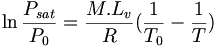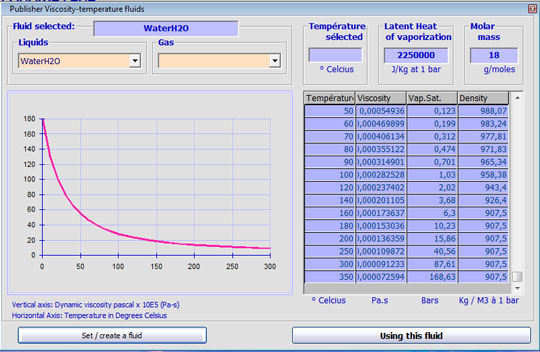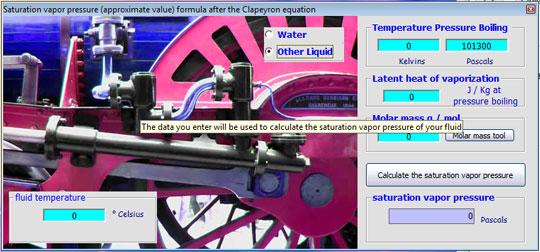The saturation vapor pressure is the pressure at which fluid passes from the gaseous state to the liquid state (or liquid to gas) at a given temperature.

If the temperature of the fluid increases, the pressure at which the fluid changes from liquid to gas (saturated vapor pressure) increases.Thus, a liquid such as water can turn into vapor at ambient pressure by supplying heat, but it is possible to make this change, without changing the temperature, by lowering the ambient pressure below the saturated vapor pressure.

When a liquid is sucked into a duct, it creates a depression, If this pressure drop lowers the fluid pressure below the saturation vapor pressure, the liquid begins to boil. (Steam production), with hydraulic , this phenomenon is called cavitation..

Cavitation is the formation of vapor bubbles due to pressure drop. In forming the bubbles increases the volume of fluid present in the low pressure zone, which has the effect of increasing pressure in some places where the gas bubble condenses violently imploding. Shocks created by the bursting bubbles destroy the walls of the organs in contact with the fluid. A pump with cavitation, wears quickly.

## Calculating the saturated vapor pressure

The approximate calculation of the saturation vapor pressure can be done using a formula outcome of the Clapeyron equation, by assuming - among other things - that the vapor behaves as an ideal gas and the enthalpy of vaporization does not vary with temperature in the range considered.with :

• T0 : boiling temperature of the substance at a pressure P0 , in Kelvin

• Psat : saturation vapor pressure, in the same unit as P0

• M : molecular weight of the substance in kg/mol

• Lv : latent heat of vaporization of the substance in J/kg

• R : Gas constant, equal to 8,31447 in J/K/mol

• T : vapor temperature in K

For water, eg:

• M = 0,018 kg/mol

• Lv = 2,26×106 J/kg

• P0 = 1013 mbar

• T0 = 373 K

With MECAFLUX standard

if you choose as fluid, water, among the 120 fluids proposed in the editor fluid viscosity, saturation vapor pressure is automatically set according to the temperature inputdetail of the setting area of the water temperature, the saturated vapor pressure of the water appears to temperature change.

All fluids in the editor viscosity are not filled with saturated steam, you can also use the tool to calculate saturation vapor pressure: